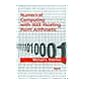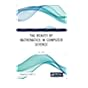Normal view MARC view ISBD view

Foundations of discrete mathematics with algorithms and programming

Material type:BookPublisher: Boca Raton CRC Press 2019Description: xvi, 518 p. Includes bibliographical references and index.ISBN: 9780815378488.DDC classification: 004.0151 Summary: Discrete Mathematics has permeated the whole of mathematics so much so it has now come to be taught even at the high school level. This book presents the basics of Discrete Mathematics and its applications to day-to-day problems in several areas. This book is intended for undergraduate students of Computer Science, Mathematics and Engineering. A number of examples have been given to enhance the understanding of concepts. The programming languages used are Pascal and C. https://www.crcpress.com/Foundations-of-Discrete-Mathematics-with-Algorithms-and-Programming/Balakrishnan-Sridharan/p/book/9780815378488
Tags from this library: No tags from this library for this title.
average rating: 0.0 (0 votes)
Item type Current location Item location Collection Call number Status Date due Barcode
Books Vikram Sarabhai Library
General Stacks
Slot 23 (0 Floor, West Wing) Non-fiction 004.0151 S7F6 (Browse shelf) Available 199354
Browsing Vikram Sarabhai Library Shelves , Shelving location: General Stacks , Collection code: Non-fiction Close shelf browser004 Z6I6-1985 Information technology: an introduction 004.0151 M9E7 Equations, models, and programs: a mathematical introduction to computer science 004.0151 O9N8 Numerical computing with IEEE floating point arithmetic 004.0151 S7F6 Foundations of discrete mathematics with algorithms and programming 004.0151 W8B3 The beauty of mathematics in computer science 004.015194 J2A7 Applied numerical methods for digital computation 004.019 D6W4 Where the action is: the foundations of embodied interaction

1. Sets, Relations and Functions.
2. Combinatorics.
3. Basics of Number Theory.
4. Introduction To Graph Theory.
5. Introduction To Algorithms.
6. Introduction To Logic.

Discrete Mathematics has permeated the whole of mathematics so much so it has now come to be taught even at the high school level. This book presents the basics of Discrete Mathematics and its applications to day-to-day problems in several areas. This book is intended for undergraduate students of Computer Science, Mathematics and Engineering. A number of examples have been given to enhance the understanding of concepts. The programming languages used are Pascal and C.

https://www.crcpress.com/Foundations-of-Discrete-Mathematics-with-Algorithms-and-Programming/Balakrishnan-Sridharan/p/book/9780815378488

There are no comments for this item.